gemseo / problems / sobieski / core

# problem module¶

The Sobieski’s SSBJ problem.

class gemseo.problems.sobieski.core.problem.SobieskiProblem(dtype='float64')[source]

Bases: object

The Sobieski’s SSBJ problem.

This problem seeks to maximize the range of a supersonic business jet (SSBJ) over a bounded design space whilst satisfying inequality constraints.

The objective and constraint functions come from a system of four disciplines:

1. the structure, computing the total aircraft mass $$y_{1,4,0}$$ and $$y_{1,2,0}$$, the fuel mass $$y_{1,4,1}$$, the wing twist $$y_{1,2,1}$$ and the five stress constraints $$g_{1,0},\ldots,g_{4,0}$$.

2. the aerodynamics discipline, computing the lift $$y_{2,1}$$, drag $$y_{2,3}$$, lift-to-drag ratio and pressure gradient constraint $$g_2$$,

3. the propulsion, computing the engine mass $$y_{3,1,0}$$, the engine scale factor $$y_{3,2,0}$$, which is also the constraint $$g_{3,0}$$, the specific fuel consumption $$y_{3,4,0}$$, the engine temperature constraint $$g_{3,1}$$, and the throttle setting constraint $$g_{3,2}$$

4. the mission, computing the range $$y_{4,0}$$.

Notes

• The structure, aerodynamics, propulsion and mission disciplines are numbered from 1 to 4.

• The variable $$y_{i,j,k}$$ is a coupling variable from the discipline $$i$$ to the discipline $$j$$.

• The aerodynamics, structure and propulsion disciplines are strongly coupled, i.e. each of them depends directly or indirectly on the others, and provide inputs to the mission discipline.

The design variables can be classified into four groups:

• the design variables which are inputs to at least two disciplines,

• $$x_{0,0}$$: the thickness-to-chord ratio,

• $$x_{0,1}$$: the altitude (ft),

• $$x_{0,2}$$: the Mach number,

• $$x_{0,3}$$: the aspect ratio,

• $$x_{0,4}$$: the wing sweep (deg),

• $$x_{0,5}$$: the wing surface area (ft 2),

• the design variables which are inputs of the structure discipline only:

• $$x_{1,0}$$: the wing taper ratio,

• $$x_{1,1}$$: the wingbox x-sectional area

• the design variables which are inputs of the aerodynamics discipline only:

• $$x_{2,0}$$: the skin friction coefficient,

• the design variables which are inputs of the propulsion discipline only:

• $$x_{3,0}$$: the throttle setting (engin mass flow).

Lastly, this problem is based on five constants:

• $$c_0$$: the minimum fuel weight,

• $$c_1$$: the miscellaneous weight,

• $$c_2$$: the maximum load factor,

• $$c_3$$: the reference engine weight,

• $$c_4$$: the minimum drag coefficient.

Parameters:

dtype (str) –

The data type for the NumPy arrays, either “float64” or “complex128”.

By default it is set to “float64”.

get_bounds_by_name(variables_names)[source]

Return the lower and upper bounds of variables.

Parameters:

variables_names (Sequence[str]) – The names of the variables.

Returns:

The lower and upper bounds of the variables; the array components keep the order of the variables.

Return type:
get_constraints(design_vector, true_cstr=False)[source]

Compute all the constraints.

Parameters:
• design_vector (ndarray) – The design vector.

• true_cstr (bool) –

If True, return the value of the constraint outputs. Otherwise, return the distance to the corresponding constraint thresholds.

By default it is set to False.

Returns:

The value of the constraints $$g_1$$, $$g_2$$ and $$g_3$$.

Return type:
get_default_inputs(names=None)[source]

Return the default variable values at the default initial point.

Parameters:

names (str | Iterable[str] | None) – The names of the variables of interest. If None, use all the variables of the problem.

Returns:

The default values of some variables at the default initial point.

Return type:

dict[str, ndarray]

get_default_inputs_equilibrium(names=None)[source]

Return the default variable values at a multidisciplinary feasible point.

The coupling variables are at the equilibrium, in the sense of the multidisciplinary analysis (MDA).

Parameters:

names (str | Iterable[str] | None) – The names of the variables of interest. If None, use all the variables of the problem.

Returns:

The default values of some variables at a multidisciplinary feasible point.

Return type:

dict[str, ndarray]

get_default_inputs_feasible(names=None)[source]

Return the default variable values at the default initial feasible point.

Parameters:

names (str | Iterable[str] | None) – The names of the variables of interest. If None, use all the variables of the problem.

Returns:

The default values of some variables at the default initial feasible point.

Return type:

dict[str, ndarray]

get_random_input(names=None, seed=None)[source]

Return a randomized starting point related to some input variables.

Parameters:
• names (str | Iterable[str] | None) – The names of the variables. If None, use all the input variables.

• seed (int | None) – The seed for the random number generation. If None, do not set the seed.

Returns:

The randomized starting point.

Return type:

ndarray

get_sobieski_constraints(g_1, g_2, g_3, true_cstr=False)[source]

Return either the value of the constraints or the distance to the thresholds.

Parameters:
• g_1 (ndarray) – The constraints from the structure discipline: g_1 to g_1 are the stresses on wing and g_1 is the wing twist.

• g_2 (ndarray) – The constraint (pressure gradient) from the aerodynamics discipline.

• g_3 (ndarray) –

The constraints from the propulsion discipline: g_3 is the engine scale factor,

g_3 is the engine temperature and g_3 is the throttle setting constraint.

• true_cstr (ndarray) –

If True, return the value of the outputs. Otherwise, return the distance to the corresponding constraint thresholds.

By default it is set to False.

Returns:

The constraints or the distance to the thresholds, according to true_cstr.

Return type:

ndarray

get_x0_feasible(names=None)[source]

Return a feasible starting point related to some input variables.

Parameters:

names (str | Iterable[str] | None) – The names of the variables. If None, use all the input variables.

Returns:

The feasible starting point.

Return type:

ndarray

normalize_inputs(input_vector)[source]

Normalize an input vector with respect to the variable bounds.

Parameters:

input_vector (ndarray) – The input vector.

Returns:

The normalized input vector with components in $$[0,1]$$.

Return type:

ndarray

unnormalize_inputs(input_vector)[source]

Unnormalize an input vector with respect to the variable bounds.

Parameters:

input_vector (ndarray) – The normalized input vector.

Returns:

The input vector in the variable space.

Return type:

ndarray

CONTRAINTS_NAMES = ('Stress_x1', 'Stress_x2', 'Stress_x3', 'Stress_x4', 'Stress_x5', 'Twist', 'Pgrad', 'ESF', 'Temperature', 'Throttle')
CONTRAINTS_NAMES_INEQUALITY = ('c_Stress_x1', 'c_Stress_x2', 'c_Stress_x3', 'c_Stress_x4', 'c_Stress_x5', 'c_Twist_upper', 'c_Twist_lower', 'c_Pgrad', 'c_ESF_upper', 'c_ESF_lower', 'c_Throttle', 'c_Temperature')
COUPLING_VARIABLES_NAMES = ('Total weight', 'Fuel weight', 'Wing twist', 'Lift', 'Drag', 'Lift/Drag', 'SFC', 'Engine weight')
DV_NAMES = ('TaperRatio', 'SectionalArea', 'Cf', 'Throttle_setting', 'eta', 'h', 'Mach', 'AR', 'Phi', 'sref')
DV_NAMES_NORMALIZED = ('x_TaperRatio', 'x_SectionalArea', 'x_Cf', 'x_Throttle_setting', 'x_eta', 'x_h', 'x_Mach', 'x_AR', 'x_Phi', 'x_sref')
ESF_LOWER_LIMIT = 0.5
ESF_UPPER_LIMIT = 1.5
STRESS_LIMIT = 1.09
TEMPERATURE_LIMIT = 1.02
TWIST_LOWER_LIMIT = 0.8
TWIST_UPPER_LIMIT = 1.04
USE_ORIGINAL_DESIGN_VARIABLES_ORDER: ClassVar[bool] = False

Whether to sort the DesignSpace as in [SSAJr98].

If so, the order of the design variables will be "x_1", "x_2", "x_3" and "x_shared". Otherwise, "x_shared", "x_1", "x_2" and "x_3".

property aerodynamics: SobieskiAerodynamics

The aerodynamics discipline.

property constants: ndarray

The constant vector.

property design_bounds: tuple[numpy.ndarray, numpy.ndarray]

The lower and upper bounds of the design variables.

property design_space: DesignSpace

The design space.

property design_space_with_physical_naming: DesignSpace

The design space with physical naming.

property initial_design: ndarray

The initial design $$x$$.

property mission: SobieskiMission

The mission discipline.

property optimum_design: ndarray

The optimal design vector found by Sobieski with BLISS.

property optimum_range: ndarray

The optimal range found by Sobieski with BLISS.

property propulsion: SobieskiPropulsion

The propulsion discipline.

property structure: SobieskiStructure

The structure discipline.

## Examples using SobieskiProblem¶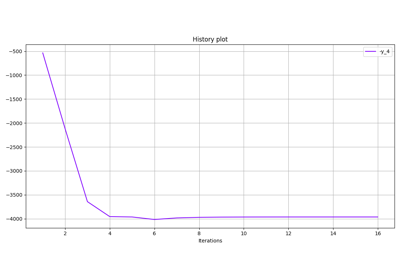Basic history

Basic history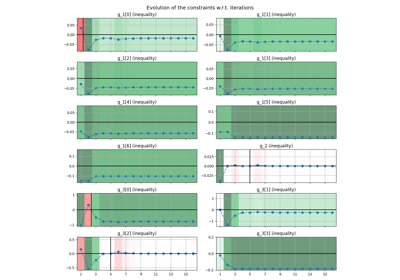Constraints history

Constraints history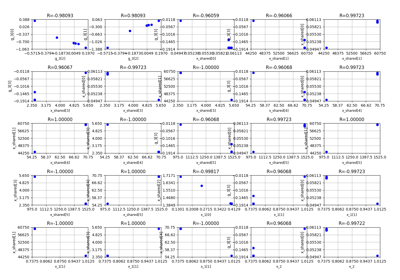Correlations

Correlations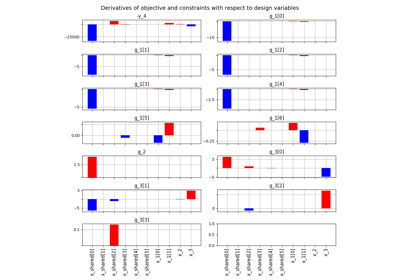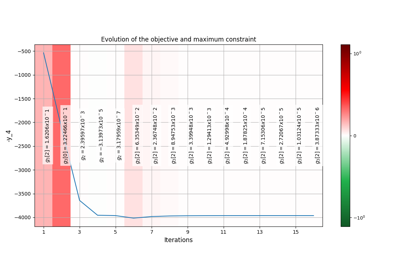Objective and constraints history

Objective and constraints history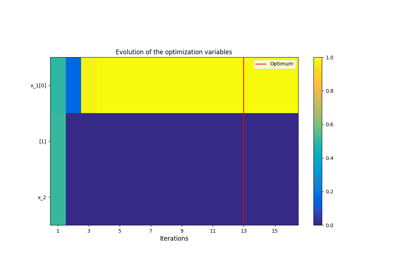Optimization History View

Optimization History View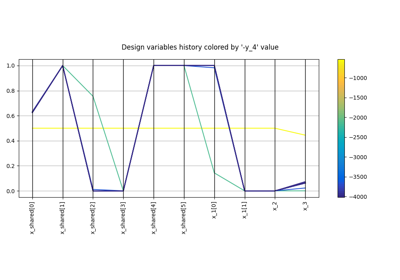Parallel coordinates

Parallel coordinates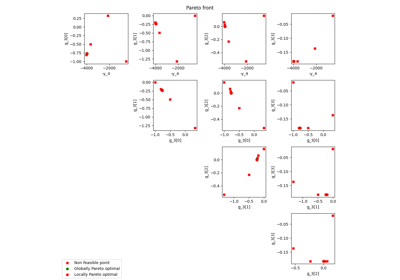Pareto front

Pareto front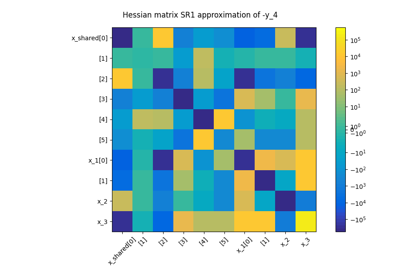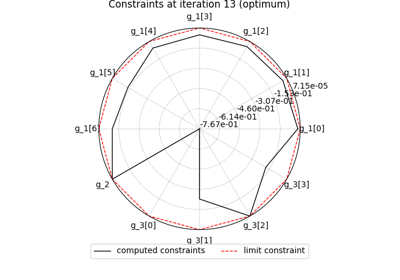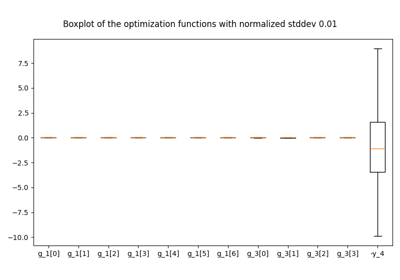Robustness

Robustness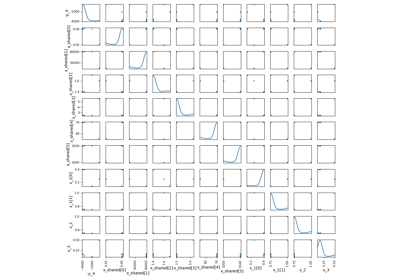Scatter plot matrix

Scatter plot matrix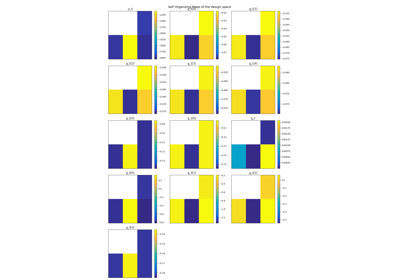Self-Organizing Map

Self-Organizing Map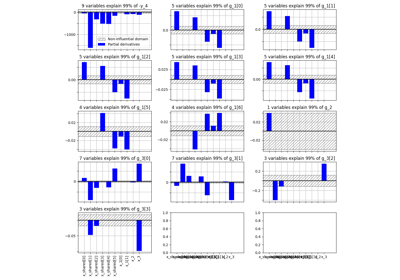Variables influence

Variables influence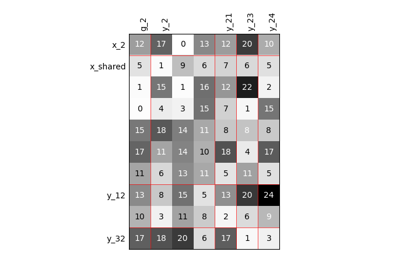Scalable diagonal discipline

Scalable diagonal disciplinePlug a surrogate discipline in a Scenario

Plug a surrogate discipline in a Scenario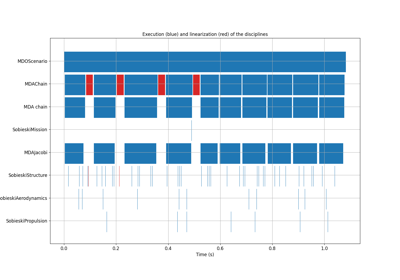Gantt Chart

Gantt Chart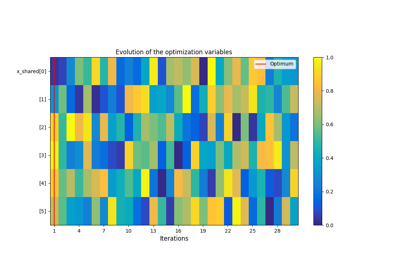BiLevel-based DOE on the Sobieski SSBJ test case

BiLevel-based DOE on the Sobieski SSBJ test case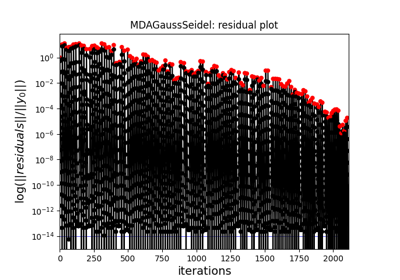BiLevel-based MDO on the Sobieski SSBJ test case

BiLevel-based MDO on the Sobieski SSBJ test case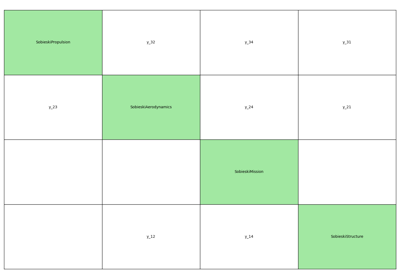IDF-based MDO on the Sobieski SSBJ test case

IDF-based MDO on the Sobieski SSBJ test caseMDF-based DOE on the Sobieski SSBJ test case

MDF-based DOE on the Sobieski SSBJ test caseMDF-based MDO on the Sobieski SSBJ test case

MDF-based MDO on the Sobieski SSBJ test case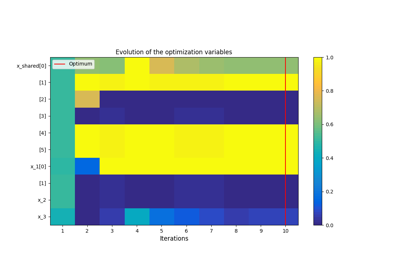Application: Sobieski’s Super-Sonic Business Jet (MDO)

Application: Sobieski's Super-Sonic Business Jet (MDO)Simple disciplinary DOE example on the Sobieski SSBJ test case

Simple disciplinary DOE example on the Sobieski SSBJ test caseExample for exterior penalty applied to the Sobieski test case.

Example for exterior penalty applied to the Sobieski test case.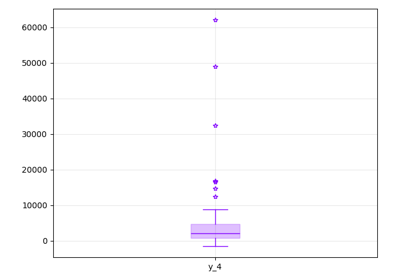Empirical estimation of statistics

Empirical estimation of statistics